CoCalc Shared FilesCDS-102 / Lab Week 10 - Statistical inference of North Carolina birth records / CDS-102 Lab Week 10 Workbook.html
Views : 6
Description: Jupyter html version of CDS-102/Lab Week 10 - Statistical inference of North Carolina birth records/CDS-102 Lab Week 10 Workbook.ipynb
CDS-102 Lab Week 10 Workbook

# CDS-102: Lab 10 Workbook¶

## Helena Gray¶

### April 6, 2017¶

In :
# Run this code block to load the Tidyverse package
.libPaths(new = "~/Rlibs")
library(tidyverse)
# Load inference() function from file "inference.RData"

Loading tidyverse: ggplot2
Conflicts with tidy packages ---------------------------------------------------
filter(): dplyr, stats
lag():    dplyr, stats

In :
nc.data<-read.csv("nc.csv")


The code below generates a full summary statistics report by running the summary() function on the dataset.

In :
summary(nc.data)

      fage            mage            mature        weeks             premie
Min.   :14.00   Min.   :13   mature mom :133   Min.   :20.00   full term:846
1st Qu.:25.00   1st Qu.:22   younger mom:867   1st Qu.:37.00   premie   :152
Median :30.00   Median :27                     Median :39.00   NA's     :  2
Mean   :30.26   Mean   :27                     Mean   :38.33
3rd Qu.:35.00   3rd Qu.:32                     3rd Qu.:40.00
Max.   :55.00   Max.   :50                     Max.   :45.00
NA's   :171                                    NA's   :2
visits            marital        gained          weight
Min.   : 0.0   married    :386   Min.   : 0.00   Min.   : 1.000
1st Qu.:10.0   not married:613   1st Qu.:20.00   1st Qu.: 6.380
Median :12.0   NA's       :  1   Median :30.00   Median : 7.310
Mean   :12.1                     Mean   :30.33   Mean   : 7.101
3rd Qu.:15.0                     3rd Qu.:38.00   3rd Qu.: 8.060
Max.   :30.0                     Max.   :85.00   Max.   :11.750
NA's   :9                        NA's   :27
lowbirthweight    gender          habit          whitemom
low    :111    female:503   nonsmoker:873   not white:284
not low:889    male  :497   smoker   :126   white    :714
NA's     :  1   NA's     :  2



The code below filters out entries in the data set with 'NA' values for the 'habit' variable.

In :
nc.data<-filter(nc.data,habit!='NA')
nc.data.nonsmoker<-filter(nc.data,habit=='nonsmoker')
nc.data.smoker<-filter(nc.data,habit=='smoker')


The code below plots a (frequency) histogram of the habit and weight variables in the dataset. The code plots both histograms on the same chart using the geom_histogram() function including inputs position="identity" and alpha=0.3 so that the plot is readable.

The code saves the histogram to a .png file using the ggsave() function.

In :
options(repr.plot.width = 9, repr.plot.height = 4)
both_hist<-ggplot(nc.data) + geom_histogram(mapping = aes(x = weight, y = ..density.., fill = habit), binwidth = 1, position = "identity", alpha = 0.3)
ggsave("both_hist.png", plot = both_hist, device="png", scale=1, width=5, height=4)
both_hist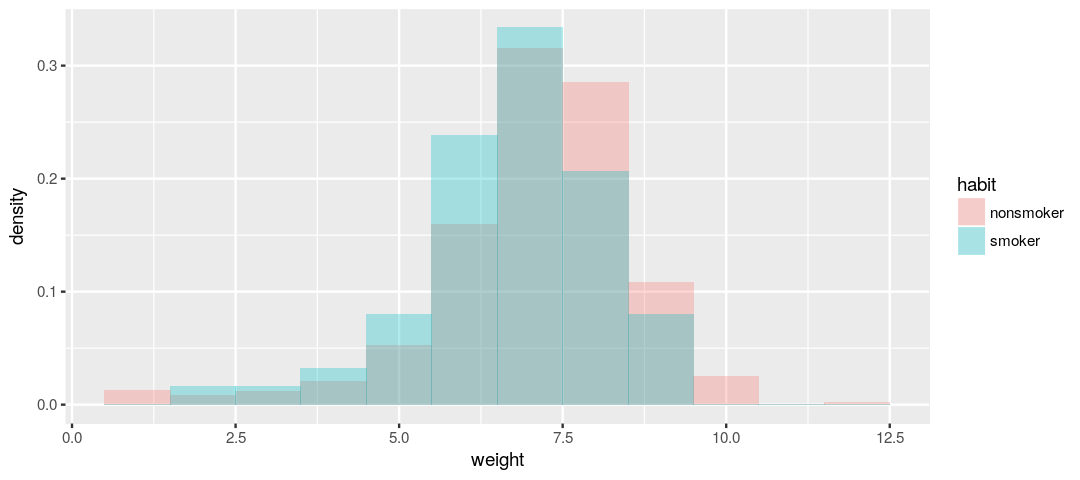The code below uses the summary() function on each of the 'habit' subsets to calculate the mean and standard deviation of birth weights for the group of non-smokers and the group of smokers. The smoking group does have a slightly lower mean birth weight. This may suggest a correlation between smoking and birth weight. We will find if this difference is statistically significant in later steps.

In :
stat.table.nonsmoker<-summarise(nc.data.nonsmoker,
mean=mean(weight), sd=sd(weight))
stat.table.nonsmoker

stat.table.smoker<-summarise(nc.data.smoker,
mean=mean(weight), sd=sd(weight))
stat.table.smoker

meansd
7.1442731.518681
meansd
6.828731.38618

For this task, the null and alternative hypotheses are specified for testing if the average weights of babies born to smoking and non-smoking mothers are different. They are shown below.

H0: There is no difference in the mean birth weights between babies born to smokers and babies born to non-smokers.

Ha: There is a difference in the mean birth weights between babies born to smokers and babies born to non-smokers.

The code below uses the inference() function to test the hypotheses specified in the previous step. The inference() function simplifies the hypothesis testing procedure, effectively hiding the manual computational work needed to perform them. For the code below, the mean birth weight is estimated for both smoking and non-smoking mothers and the type of test is specified as a 'hypothesis test'. The test is based on the Central Limit Theorem which is specified by the parameter 'method' and setting it to 'theoretical'.

In :
inference(y = weight, x = habit, data = nc.data,
statistic = "mean", type = "ht", null = 0,
alternative = "twosided", method = "theoretical",
order = c("smoker", "nonsmoker"))

Response variable: numerical
Explanatory variable: categorical (2 levels)
n_smoker = 126, y_bar_smoker = 6.8287, s_smoker = 1.3862
n_nonsmoker = 873, y_bar_nonsmoker = 7.1443, s_nonsmoker = 1.5187
H0: mu_smoker =  mu_nonsmoker
HA: mu_smoker != mu_nonsmoker
t = -2.359, df = 125
p_value = 0.0199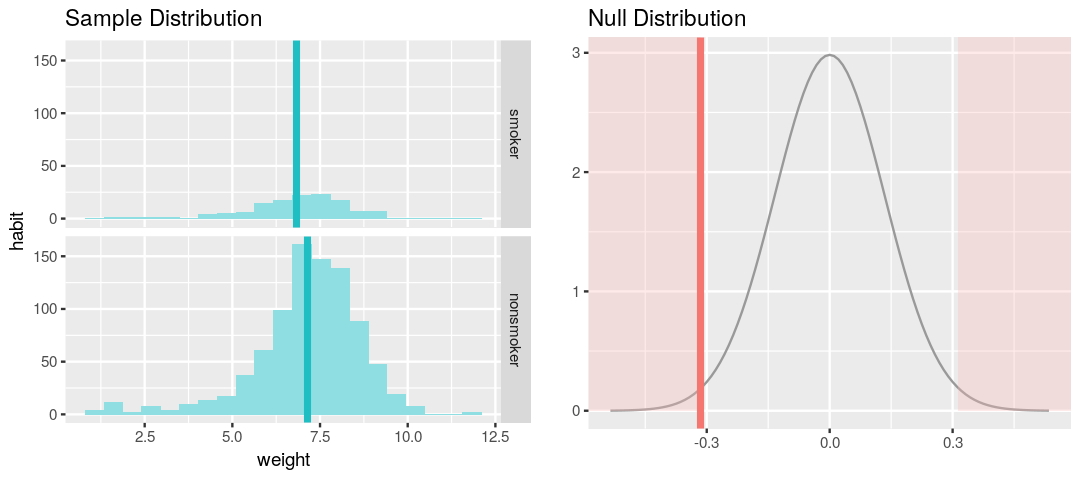The results of the hypothesis test produce a p-value that is less than the significance level which is .05, meaning that the null hypothesis, that there is no statistically significant difference between smokers and nonsmokers, can be rejected.

The code below uses the inference() function again but this time the 'type' parameter is changed to 'ci' to produce a confidence interval. The 'null' and 'alternative' inputs are removed becaue the confidence interval will indicate a non-rejection of the null hypothesis if the value 0 is included within the confidence interval (this suggests that there is literally zero difference between the true means of both populations). The confidence interval indicates the range of values in which the difference between the true means of both populations lies.

In :
inference(y = weight, x = habit, data = nc.data,
statistic = "mean", type = "ci",
method = "theoretical",
order = c("smoker", "nonsmoker"))

Response variable: numerical, Explanatory variable: categorical (2 levels)
n_smoker = 126, y_bar_smoker = 6.8287, s_smoker = 1.3862
n_nonsmoker = 873, y_bar_nonsmoker = 7.1443, s_nonsmoker = 1.5187
95% CI (smoker - nonsmoker): (-0.5803 , -0.0508)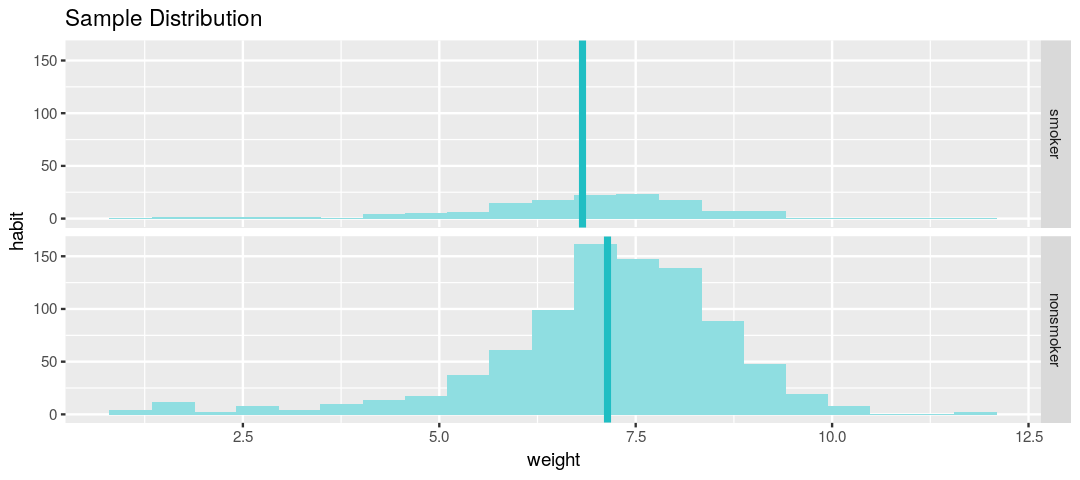In this example, both values of the confidence interval are negative, indicating that difference of the second mean from the first mean is negative, meaning that the second population (nonsmokers) have a higher mean birth weight.

The code below calculates a 95% confidence interval for the average length of pregnancies (weeks variable). Rather than finding a difference between true means of true populations, this confidence interval just finds the range of values in which the true mean of the variable for the population of interest lies (in this case it is the true mean of the length of pregnancy in weeks for the entire population of women in North Carolina).

In :
inference(y = weeks, data = nc.data,
statistic = "mean", type = "ci",
method = "theoretical",
order = c("smoker", "nonsmoker"))

Single numerical variable
n = 998, y-bar = 38.3347, s = 2.9316
95% CI: (38.1526 , 38.5168)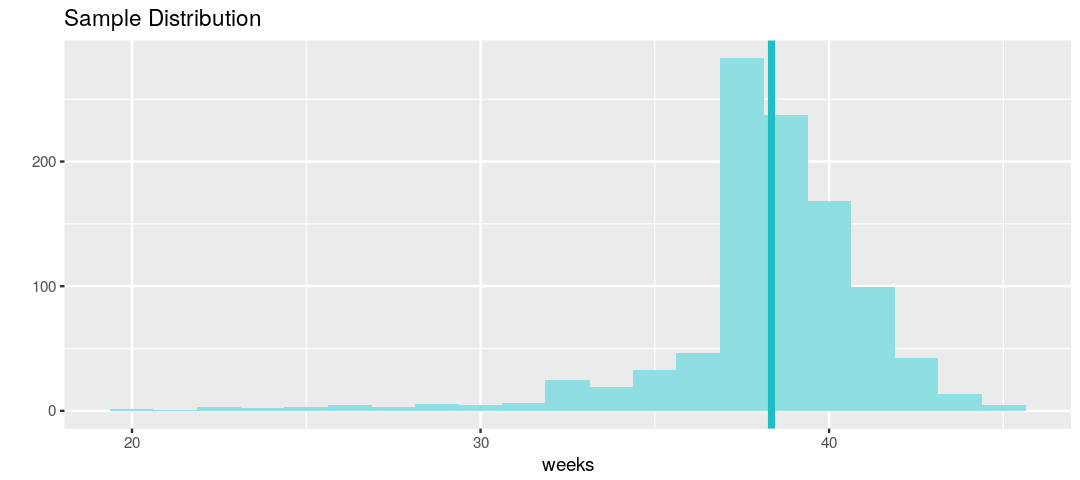The results indicate that the true mean of the length of pregnancy for women in North Carolina is somewhere between 38.1 weeks and 38.5 weeks.

The code below calculates a new confidence interval for the same parameter at the 90% confidence level. The confidence level is changed by adding a new argument to the function: conf_level = 0.90.

In :
inference(y = weeks, data = nc.data,
statistic = "mean", type = "ci",
method = "theoretical",
order = c("smoker", "nonsmoker"),conf_level=.90)

Single numerical variable
n = 998, y-bar = 38.3347, s = 2.9316
90% CI: (38.1819 , 38.4874)This change in confidence level narrows the range of values containing the true mean of the average length of pregnancy for women in North Carolina.

The code below tests the hypothesis that there is no difference in mean number of hospital visits for pregnant married women and pregnant unmarried women. The code below uses the inference() function to perform a hypothesis test and a confidence interval using an α level of .05. This defines both the p-value threshold for statistical significance and the size of the confidence interval.

## Do married mothers tend to visit the hospital more than single mothers?¶

### Ha: There is a difference in mean number of visits to hospital between married and unmarried mothers.¶

#### alpha level = .05¶

In :
inference(y = visits, x = marital, data = nc.data,
statistic = "mean", type = "ht", null = 0,
alternative = "twosided", method = "theoretical",
order = c("married", "unmarried"))

Response variable: numerical
Explanatory variable: categorical (2 levels)
n_married = 380, y_bar_married = 10.9553, s_married = 4.2408
n_not married = 611, y_bar_not married = 12.82, s_not married = 3.5883
H0: mu_married =  mu_not married
HA: mu_married != mu_not married
t = -7.1298, df = 379
p_value = < 0.0001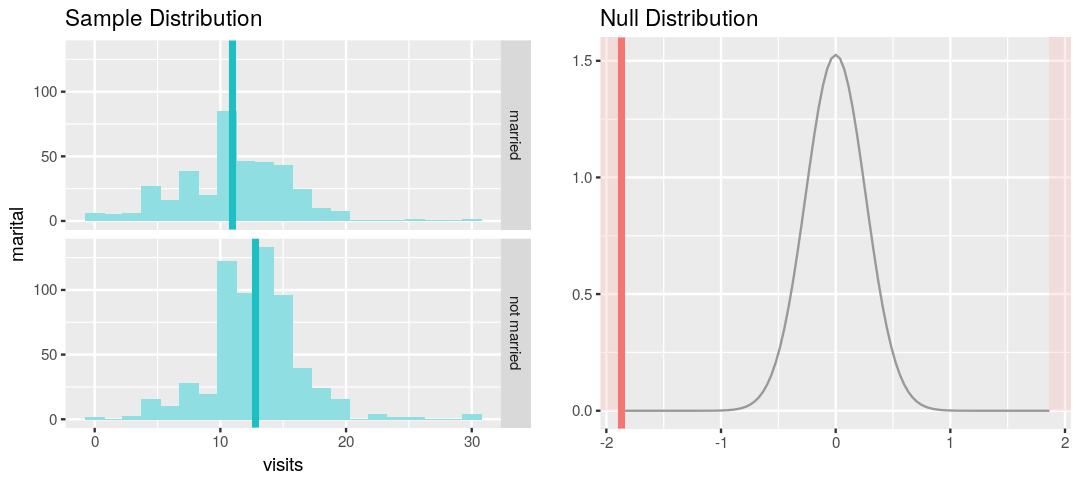In :
inference(y = visits, x = marital, data = nc.data,
statistic = "mean", type = "ci",
method = "theoretical",
order = c("married", "unmarried"))

Response variable: numerical, Explanatory variable: categorical (2 levels)
n_married = 380, y_bar_married = 10.9553, s_married = 4.2408
n_not married = 611, y_bar_not married = 12.82, s_not married = 3.5883
95% CI (married - not married): (-2.3789 , -1.3505)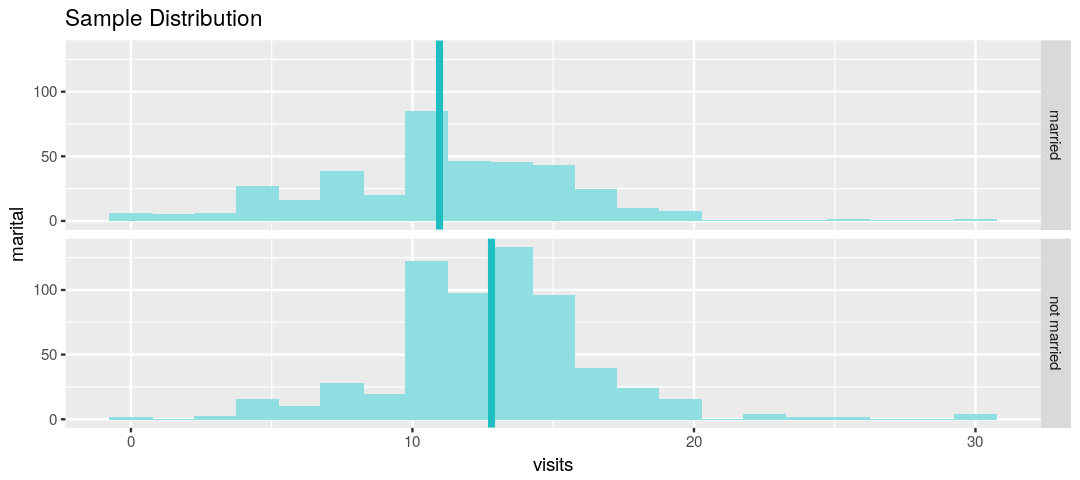In [ ]: